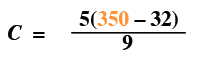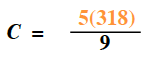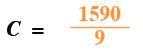### Home > CC2 > Chapter 4 > Lesson 4.2.3 > Problem4-52

4-52.

Paige traveled to Australia and is making her favorite bread recipe. She usually bakes the bread in a $350º\text{F}$ oven. She is surprised to learn that the oven temperatures in Australia are measured in degrees Celsius. Using the formula below and the Order of Operations, help Paige determine how she should set the oven in degrees Celsius. Homework Help ✎

$C=\frac{5(F-32)}{9}$

You are given degrees Fahrenheit and you need degrees Celsius.

Substitute $350º\text{F}$ for the variable $\text{F}$. Then solve within the parentheses.

Simplify the numerator by multiplying.

Divide to get your answer.